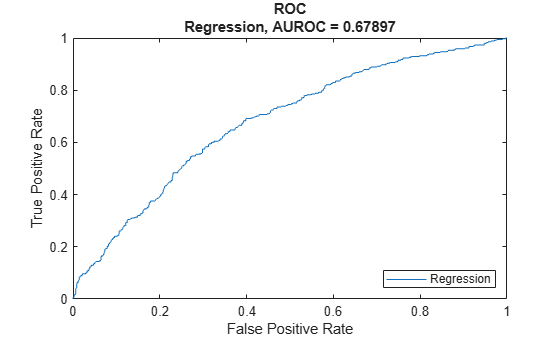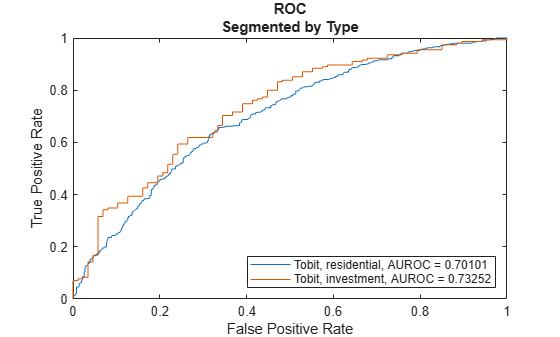modelDiscriminationPlot

Plot ROC curve

Description

example

modelDiscriminationPlot(lgdModel,data) generates the receiver operating characteristic (ROC) curve. modelDiscriminationPlot supports segmentation and comparison against a reference model.

example

modelDiscriminationPlot(___,Name,Value) specifies options using one or more name-value pair arguments in addition to the input arguments in the previous syntax.

example

h = modelDiscriminationPlot(ax,___,Name,Value) specifies options using one or more name-value pair arguments in addition to the input arguments in the previous syntax and returns the figure handle h.

Examples

collapse all

This example shows how to use fitLGDModel to fit data with a Regression model and then use modelDiscriminationPlot to plot the ROC.

Load the loss given default data.

ans=8×4 table
LTV        Age         Type           LGD
_______    _______    ___________    _________

0.89101    0.39716    residential     0.032659
0.70176     2.0939    residential      0.43564
0.72078     2.7948    residential    0.0064766
0.37013      1.237    residential     0.007947
0.36492     2.5818    residential            0
0.796     1.5957    residential      0.14572
0.60203     1.1599    residential     0.025688
0.92005    0.50253    investment      0.063182

Partition Data

Separate the data into training and test partitions.

rng('default'); % for reproducibility
NumObs = height(data);

c = cvpartition(NumObs,'HoldOut',0.4);
TrainingInd = training(c);
TestInd = test(c);

Create a Regression LGD Model

Use fitLGDModel to create a Regression model using training data.

lgdModel = fitLGDModel(data(TrainingInd,:),'regression');
disp(lgdModel)
Regression with properties:

ResponseTransform: "logit"
BoundaryTolerance: 1.0000e-05
ModelID: "Regression"
Description: ""
UnderlyingModel: [1x1 classreg.regr.CompactLinearModel]
PredictorVars: ["LTV"    "Age"    "Type"]
ResponseVar: "LGD"

Display the underlying model.

disp(lgdModel.UnderlyingModel)
Compact linear regression model:
LGD_logit ~ 1 + LTV + Age + Type

Estimated Coefficients:
Estimate       SE        tStat       pValue
________    ________    _______    __________

(Intercept)        -4.7549      0.36041    -13.193    3.0997e-38
LTV                 2.8565      0.41777     6.8377    1.0531e-11
Age                -1.5397     0.085716    -17.963    3.3172e-67
Type_investment     1.4358       0.2475     5.8012     7.587e-09

Number of observations: 2093, Error degrees of freedom: 2089
Root Mean Squared Error: 4.24
F-statistic vs. constant model: 181, p-value = 2.42e-104

Plot ROC Data

Use modelDiscriminationPlot to plot the ROC for the test data set.

modelDiscriminationPlot(lgdModel,data(TestInd,:))This example shows how to use fitLGDModel to fit data with a Tobit model and then use modelDiscriminationPlot to plot the ROC.

Load the loss given default data.

ans=8×4 table
LTV        Age         Type           LGD
_______    _______    ___________    _________

0.89101    0.39716    residential     0.032659
0.70176     2.0939    residential      0.43564
0.72078     2.7948    residential    0.0064766
0.37013      1.237    residential     0.007947
0.36492     2.5818    residential            0
0.796     1.5957    residential      0.14572
0.60203     1.1599    residential     0.025688
0.92005    0.50253    investment      0.063182

Partition Data

Separate the data into training and test partitions.

rng('default'); % for reproducibility
NumObs = height(data);

c = cvpartition(NumObs,'HoldOut',0.4);
TrainingInd = training(c);
TestInd = test(c);

Create a Tobit LGD Model

Use fitLGDModel to create a Tobit model using training data.

lgdModel = fitLGDModel(data(TrainingInd,:),'tobit');
disp(lgdModel)
Tobit with properties:

CensoringSide: "both"
LeftLimit: 0
RightLimit: 1
ModelID: "Tobit"
Description: ""
UnderlyingModel: [1x1 risk.internal.credit.TobitModel]
PredictorVars: ["LTV"    "Age"    "Type"]
ResponseVar: "LGD"

Display the underlying model.

disp(lgdModel.UnderlyingModel)
Tobit regression model:
LGD = max(0,min(Y*,1))
Y* ~ 1 + LTV + Age + Type

Estimated coefficients:
Estimate        SE         tStat       pValue
_________    _________    _______    __________

(Intercept)         0.058257      0.02728     2.1355      0.032833
LTV                  0.20126     0.031403     6.4088    1.8072e-10
Age                -0.095407    0.0072398    -13.178             0
Type_investment      0.10208     0.018048     5.6561     1.761e-08
(Sigma)              0.29288    0.0057086     51.304             0

Number of observations: 2093
Number of left-censored observations: 547
Number of uncensored observations: 1521
Number of right-censored observations: 25
Log-likelihood: -698.383

Plot ROC Data

Use modelDiscriminationPlot to plot the ROC for the test data set.

modelDiscriminationPlot(lgdModel,data(TestInd,:),"SegmentBy","Type","DiscretizeBy","median")Input Arguments

collapse all

Loss given default model, specified as a previously created Regression or Tobit object using fitLGDModel.

Data Types: object

Data, specified as a NumRows-by-NumCols table with predictor and response values. The variable names and data types must be consistent with the underlying model.

Data Types: table

(Optional) Valid axis object, specified as an ax object that is created using axes. The plot will be created in the axes specified by the optional ax argument instead of in the current axes (gca). The optional argument ax must precede any of the input argument combinations.

Data Types: object

Name-Value Arguments

Specify optional comma-separated pairs of Name,Value arguments. Name is the argument name and Value is the corresponding value. Name must appear inside quotes. You can specify several name and value pair arguments in any order as Name1,Value1,...,NameN,ValueN.

Example: modelDiscriminationPlot(lgdModel,data(TestInd,:),'DataID','Testing','DiscretizeBy','median')

Data set identifier, specified as the comma-separated pair consisting of 'DataID' and a character vector or string. The DataID is included in the output for reporting purposes.

Data Types: char | string

Discretization method for LGD data, specified as the comma-separated pair consisting of 'DiscretizeBy' and a character vector or string.

• 'mean' — Discretized response is 1 if observed LGD is greater than or equal to the mean LGD, 0 otherwise.

• 'median' — Discretized response is 1 if observed LGD is greater than or equal to the median LGD, 0 otherwise.

• 'positive' — Discretized response is 1 if observed LGD is positive, 0 otherwise (full recovery).

• 'total' — Discretized response is 1 if observed LGD is greater than or equal to 1 (total loss), 0 otherwise.

Data Types: char | string

Name of a column in the data input, not necessarily a model variable, to be used to segment the data set, specified as the comma-separated pair consisting of 'SegmentBy' and a character vector or string. One AUROC is reported for each segment, and the corresponding ROC data for each segment is returned in the optional output.

Data Types: char | string

LGD values predicted for data by the reference model, specified as the comma-separated pair consisting of 'ReferenceLGD' and a NumRows-by-1 numeric vector. The ROC curve is plotted for both the lgdModel object and the reference model.

Data Types: double

Identifier for the reference model, specified as the comma-separated pair consisting of 'ReferenceID' and a character vector or string. 'ReferenceID' is used in the plot for reporting purposes.

Data Types: char | string

Output Arguments

collapse all

Figure handle for the line objects, returned as handle object.

collapse all

Model Discrimination Plot

The modelDiscriminationPlot function plots the receiver operator characteristic (ROC) curve.

The modelDiscriminationPlot function also shows the area under the receiver operator characteristic (AUROC) curve, sometimes called simply the area under the curve (AUC). This metric is between 0 and 1 and higher values indicate better discrimination.

A numeric prediction and a binary response are needed to plot the ROC and compute the AUROC. For LGD models, the predicted LGD is used directly as the prediction. However, the observed LGD must be discretized into a binary variable. By default, observed LGD values greater than or equal to the mean observed LGD are assigned a value of 1, and values below the mean are assigned a value of 0. This discretized response is interpreted as “high LGD” vs. “low LGD.” The ROC curve and the AUROC curve measure how well the predicted LGD separates the “high LGD” vs. the “low LGD” observations. The discretization criterion can be changed with the DiscretizeBy name-value pair argument for modelDiscriminationPlot.

The ROC curve is a parametric curve that plots the proportion of

• High LGD cases with predicted LGD greater than or equal to a parameter t, or true positive rate (TPR)

• Low LGD cases with predicted LGD greater than or equal to the same parameter t, or false positive rate (FPR)

The parameter t sweeps through all the observed predicted LGD values for the given data. If the AUROC value or the ROC curve data are needed programmatically, use the modelDiscrimination function. For more information about ROC curves, see Performance Curves.

 Baesens, Bart, Daniel Roesch, and Harald Scheule. Credit Risk Analytics: Measurement Techniques, Applications, and Examples in SAS. Wiley, 2016.

 Bellini, Tiziano. IFRS 9 and CECL Credit Risk Modelling and Validation: A Practical Guide with Examples Worked in R and SAS. San Diego, CA: Elsevier, 2019.